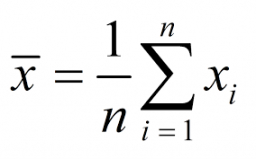# Average age

There are 20 students in the class. Several are 12 years old, and others are 13 years old. Find out how old is twelve when the average age of a class is:

a) 12.5 years

b) 12.75 years

c) 12.3 years

d) 12.35 years

a =  10
b =  5
c =  14
d =  13

### Step-by-step explanation:Did you find an error or inaccuracy? Feel free to write us. Thank you!

Tips for related online calculators
Looking for help with calculating arithmetic mean?
Looking for a statistical calculator?
Need help calculating sum, simplifying, or multiplying fractions? Try our fraction calculator.
Do you have a linear equation or system of equations and looking for its solution? Or do you have a quadratic equation?

#### Grade of the word problem:

We encourage you to watch this tutorial video on this math problem: